## Astrolabe

Understanding the Astrolabe

# The Problem

Find the time of day from the observed position of the Sun or some fixed star (i.e. not a planet nor the Moon).

# Astronomy

Although the Earth orbits around the Sun and the stars all move around too, from the point of view of an observer on the Earth the stars seem to be at fixed positions relative to each other.  This is due to the stars being extremely far away compared to their own motion and to the diameter of Earth's orbit.

For a first approximation we will therefore assume that the sky looks like a very large sphere on which the stars are fixed:  the celestial sphere.

A few objects do move on that sphere:  the planets, the Sun and the Moon.  We will not consider them here.  We will also assume that the size of the Earth is insignificant compared to the size of the celestial sphere.

## Local View

A human observer H on the surface of the Earth can view everything from the horizon upwards to the zenith, the point directly above.

H also knows the local North direction.  Using the horizon and the North, H can assign a position to each object by two coordinates:  the azimuth (number of degrees from North), and the altitude (degrees up from the horizon).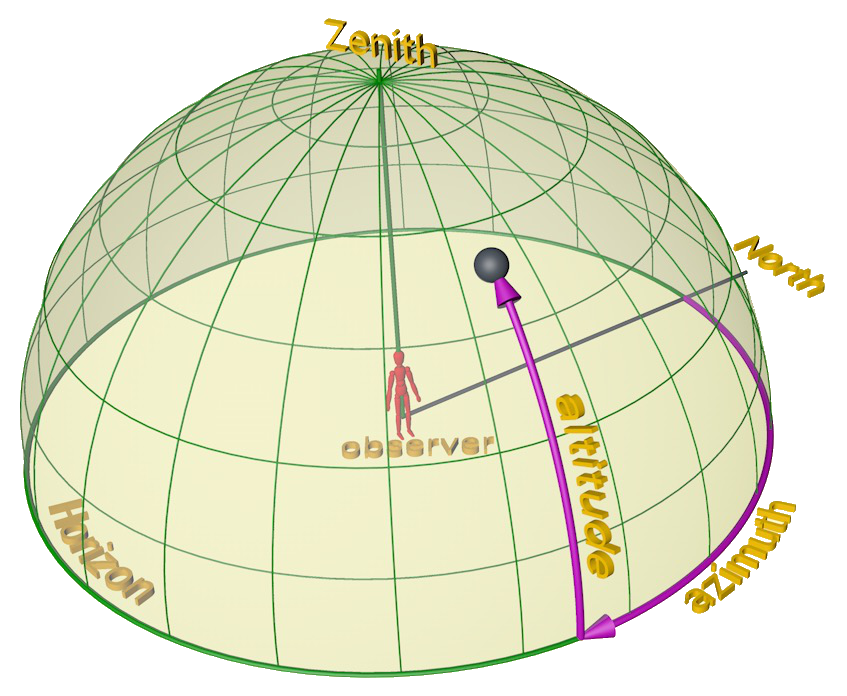Local View: azimuth and altitude.

## Celestial Sphere

The local view depends on the location of the observer on the Earth and at each moment spans only half of the entire celestial sphere.  The celestial sphere is the entire sphere, with the Earth in the centre: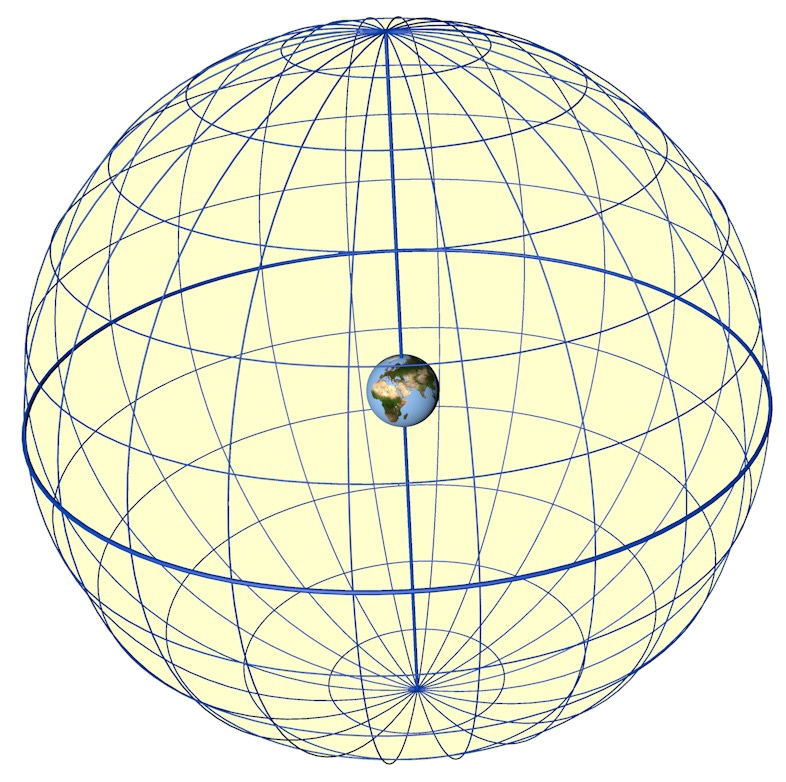the Celestial Sphere

The celestial sphere has a North and South pole and an equator that correspond to the poles and equator of Earth.  A similar coordinate system is used to locate stars and other objects.  The right ascension is measured along the equator, the declination is the number of degrees up from the equator towards the North pole.  The right ascension is the number of degrees from a point on the equator that is fixed as the vernal equinox, not important here yet.

Coordinates of objects on the celestial sphere do not depend on the time of day or on the position of the observer.

## Combining the local view with the celestial sphere

For an observer H (in the image below H is roughly in southern Europe) the local view is tilted (down and towards the reader) relative to the celestial sphere.  In addition, as the Earth rotates, the local view rotates with it.  To H this looks like the celestial sphere rotates in the other sense around a point that roughly coincides with the location of the Pole Star (in the Northern hemisphere; the Southern Cross in the Southern hemisphere).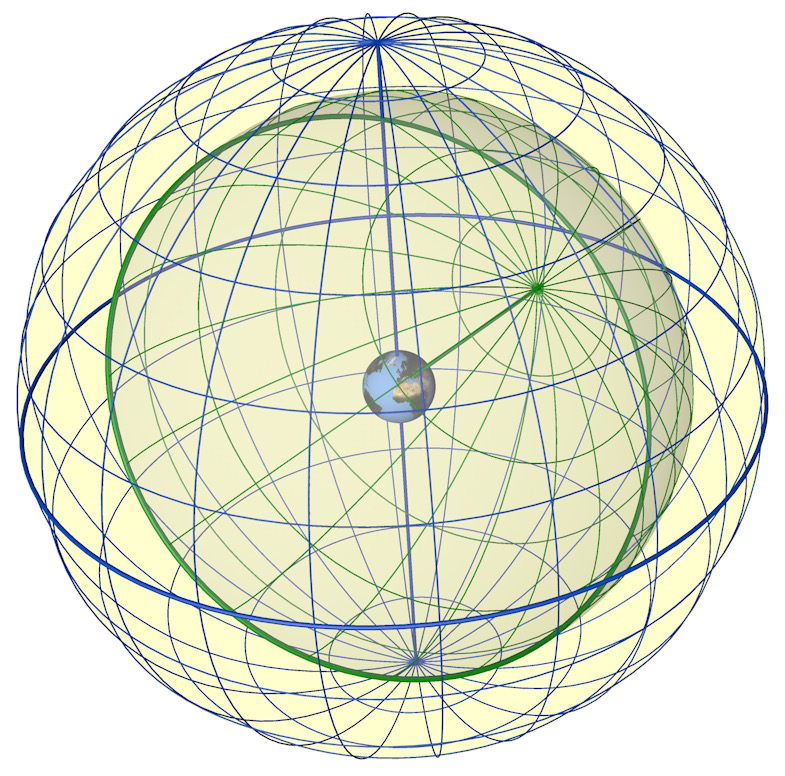Celestial and local spheres

To tell the time means to know how far the Earth has rotated, which can be deduced from how far the celestial sphere seems to have rotated, and that can be computed from the positions of stars on this sphere.  But the observer can only measure in terms of azimuth and altitude.  We need an instrument to convert between the local view coordinates and the celestial coordinates.

The conceptually easiest way to do this would be to construct two spheres, as in the image.  Locate the observed star on the celestial sphere, find the point on the local view sphere corresponding to the star's observed azimuth and altitude, then turn the local view sphere so that this point falls on the celestial position.  The angle between the two then gives the time.

However, this is clumsy for two reasons:  two concentric spheres are difficult to make, and spheres take a lot of space.

A flat instrument would be much better:  an astrolabe.

# Problem of making a flat instrument

Both spheres in the conceptual solution will have to be "flattened", but there is no easy mapping of a sphere onto a plane:  all such maps distort in some way.

# Parts

Mater — climates — rete

# Flattening the Sphere

There are many ways to map a sphere onto a plane.  Given that we want to move positions of objects in the sky  by rotating the rete, and because we want to draw onto the climates using only compass and ruler, a desirable projection would map circles to circles.

Fortunately there is such a projection:  the stereographic projection.

## The Stereographic Projection

The stereographic projection transforms points P on a sphere into points P' on a plane Z by using a centre S:  P' is the point where the line PS cuts the plane Z: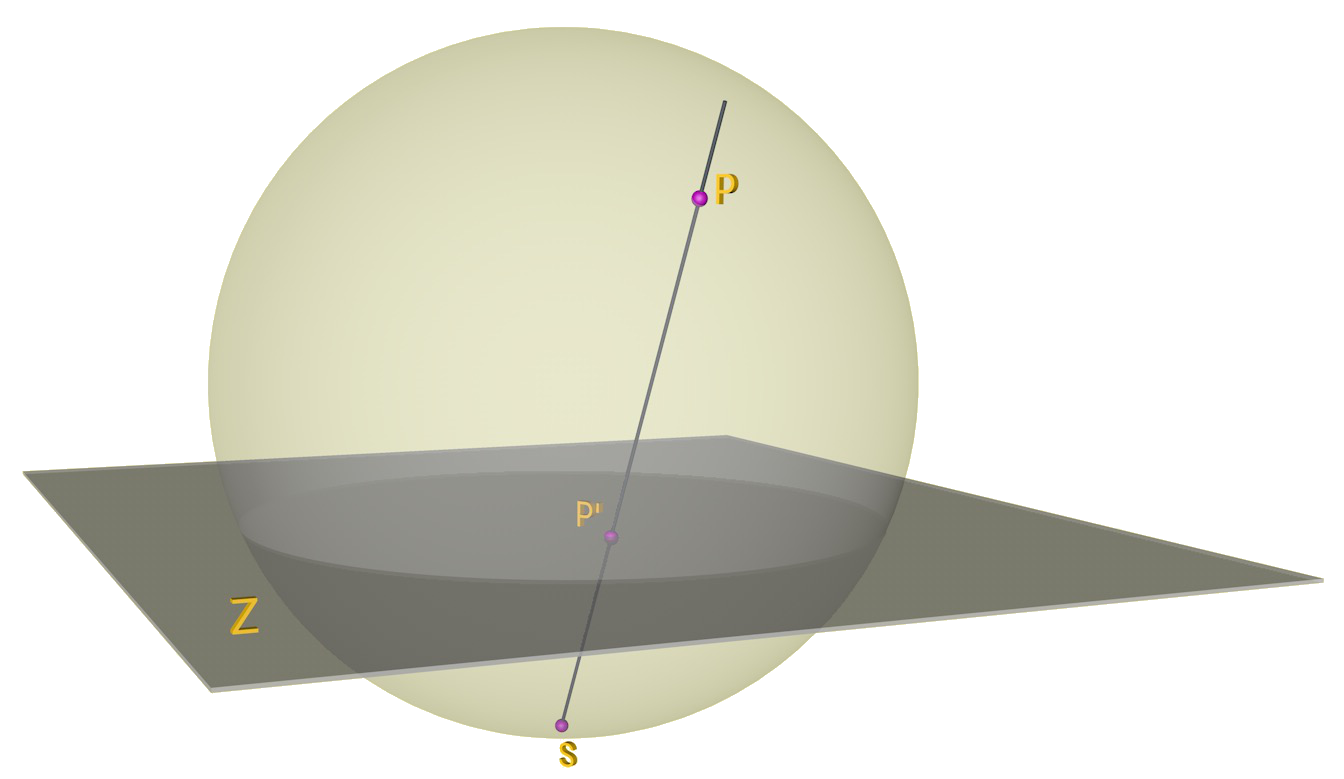Stereographic projection

For the astrolabe, the sphere is the celestial sphere (the "far away stars"), the plane is the plane of the equator and the projection centre is the South pole.

The most important property of the stereographic projection is that it transforms a circle on the sphere into a circle on the plane.  This is important because we want to project two different spheres onto the plane:  the first one is the starry sky, with the celestial North (North star approximately) at the top, and the other one is the local visible hemisphere with the zenith at the top and the local horizon as the "equator".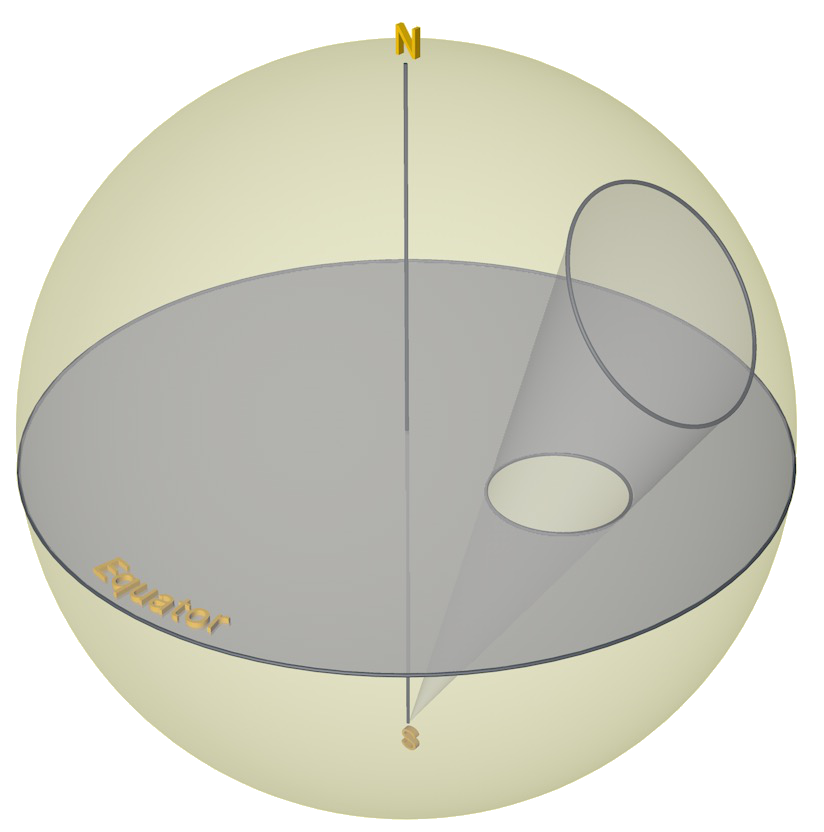Stereographic projection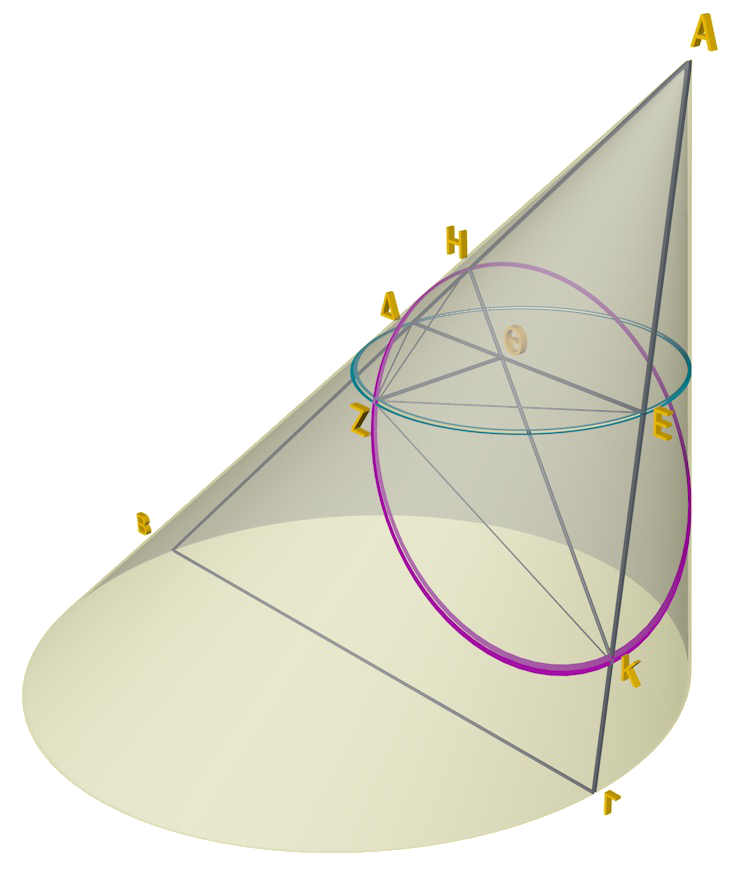Circle Theorem (3D view)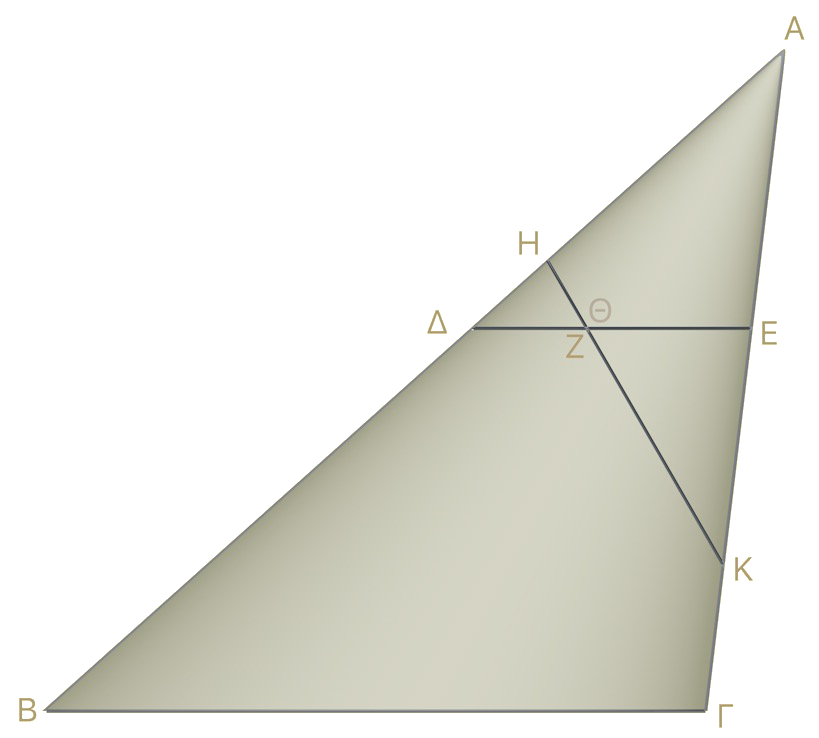Circle Theorem — in axial plane